# INTERCEPT Function Examples – Excel, VBA, & Google Sheets

This Tutorial demonstrates how to use the Excel INTERCEPT Function in Excel to calculate the y intercept.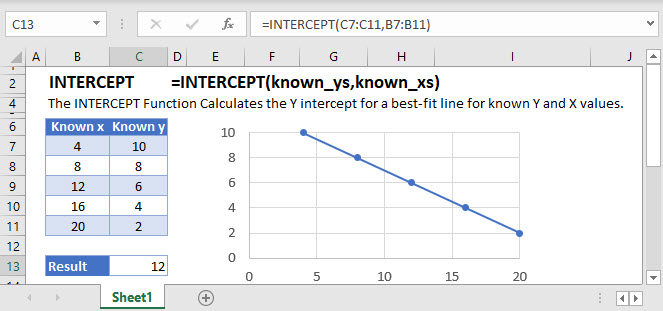## INTERCEPT Function Overview

The INTERCEPT Function Calculates the Y intercept for a best-fit line for known Y and X values.

To use the INTERCEPT Excel Worksheet Function, select a cell and type: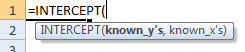(Notice how the formula inputs appear)

## INTERCEPT Function Syntax and inputs:

known_y’s – An array of known Y values.

known_x’s – An array of known X values.

## What is INTERCEPT?

The INTERCEPT function in Excel is used to calculate the y-intercept of a line given known x and y values.

The INTERCEPT function can be used in conjunction with the SLOPE function to find the equation of a linear line,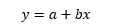Suppose I have a table of data with x and y values: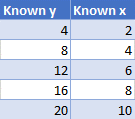The y-intercept of the line can be found by extending the of best fit backwards until it intercepts the y-axis: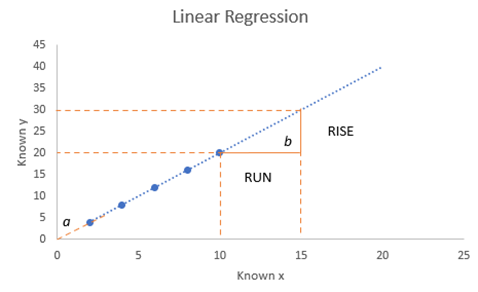Using the above chart, we find the intercept, a to be zero.

Using the INTERCEPT function: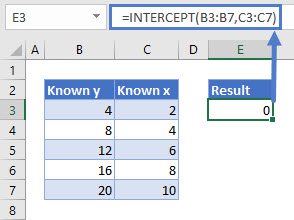## How to use INTERCEPT

The INTERCEPT function takes two arguments:

Where known_y’s and known_x’s refer to the x and y data in your data table.

Using our first example, the function is written as:

## Pairing SLOPE and INTERCEPT

To pair SLOPE and INTERCEPT, an equation can be written as:

## Interested in More Forecasting?

Pair INTERCEPT with SLOPE to see how to create the equation of a line and visit our other articles on Forecasting with Exponential Smoothing, TREND and LINEST functions.

## GROWTH function in Google Sheets

The GROWTH function works exactly the same in Google Sheets as in Excel.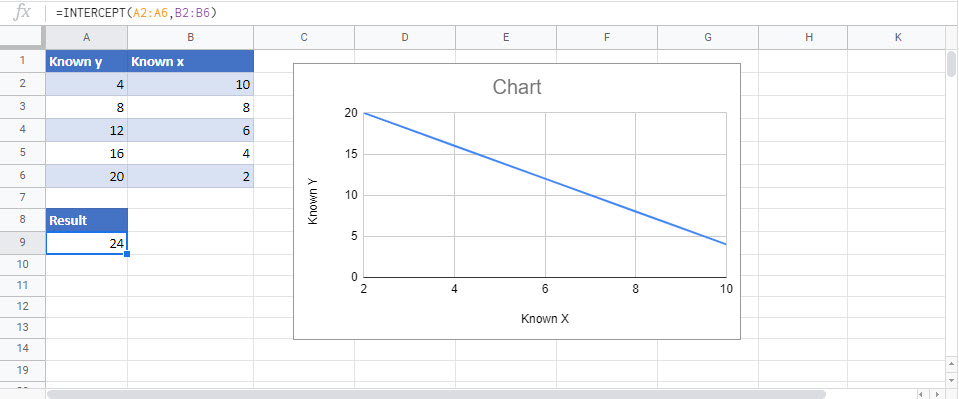## INTERCEPT Examples in VBA

You can also use the INTERCEPT function in VBA. Type:
`application.worksheetfunction.intercept(known_ys,known_xs)`
For the function arguments (known_y’s, etc.), you can either enter them directly into the function, or define variables to use instead.